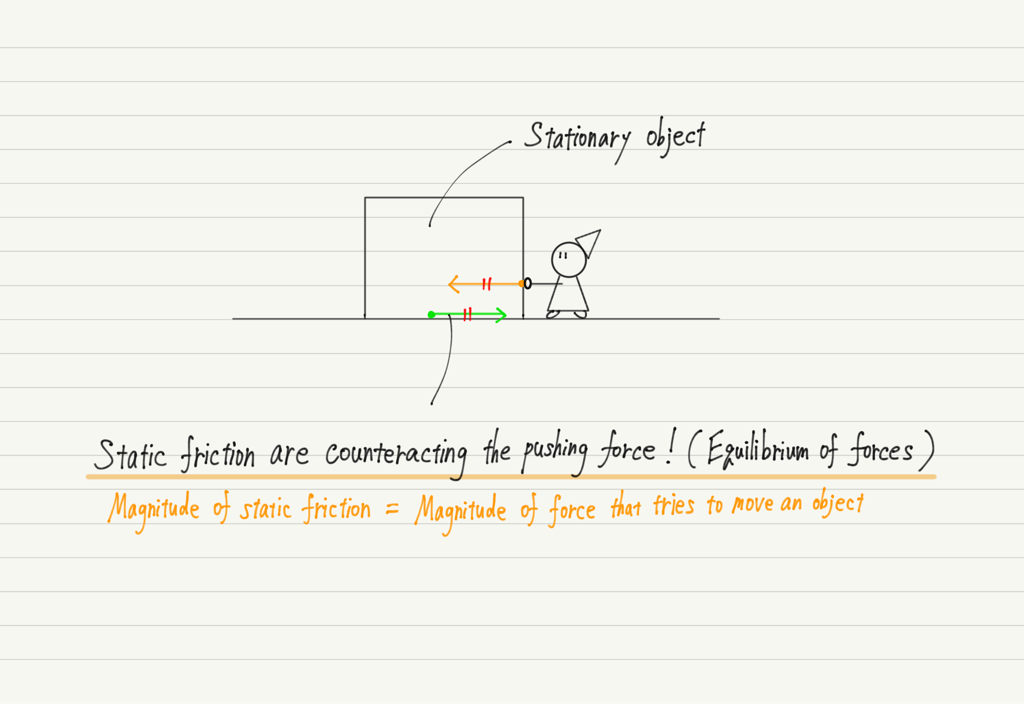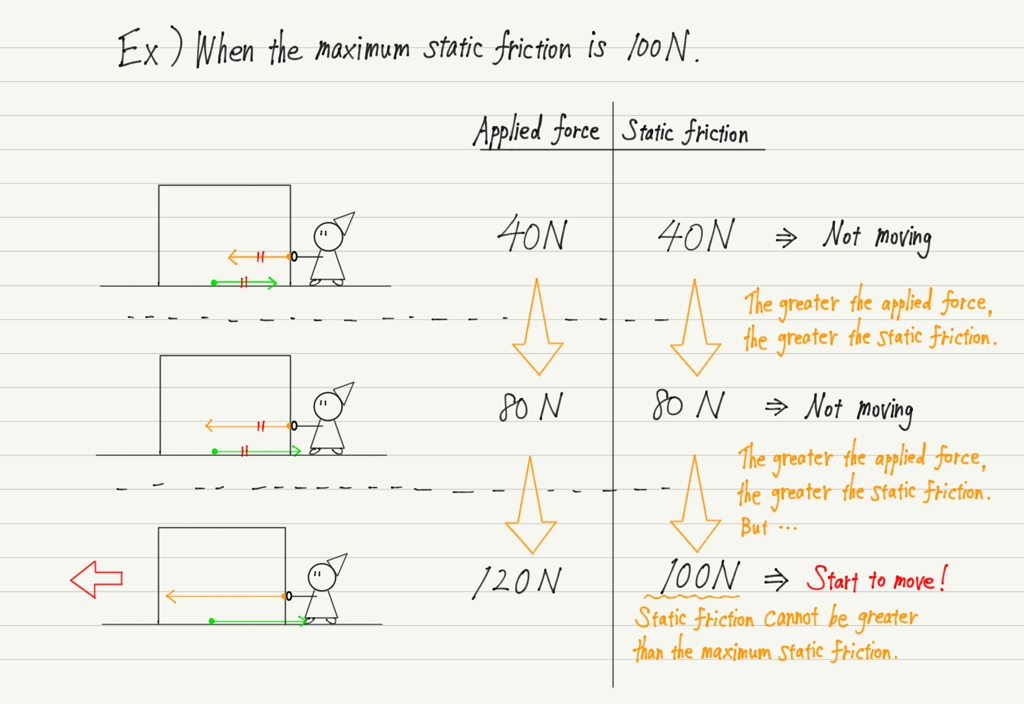# Static Friction

What do you think of when you hear the word “Frictional force”?

Is it a force that prevents an object from moving?

In fact, it is not necessarily true that “Friction = Disturbing force”.
For example, a car is driven by the friction between the ground and the tires (if the ground were smooth with zero friction, the tires would simply spin). In this case, friction is not a hindrance, but a driving force.
As can be seen from this example, frictional forces are essential for doing physics.

There are various types of friction, and in high school physics, we will study two of them: “static friction” and “kinetic friction”.
Let’s learn about static friction this time!

## Frictional force when an object does not start moving

Imagine that there is an object in front of you with enough mass that you can manage to move it if you push it hard enough. You push the object with a weak force at first, and gradually increase the force.

Have you got an image?

The object does not start moving as long as it is initially pushed with a weak force. It remains stationary.
What does it mean that the force is applied but it remains stationary?

Yes, that means frictional forces are preventing it from starting to move!

This force that prevents an object from moving is called the “Static friction”.

Now let us consider the magnitude of the static friction.

If the object does not move despite the applied force, the magnitude of the static friction force is the same as the applied force, and in the opposite direction. The static frictional force cancels the applied force.Let me give you an example with concrete numbers.

If you push with a force of 10 N and it does not move, the static friction is also 10 N.

If you push with a force of 90 N and it does not move, the static friction is also 90 N.

If you push with a force of 200 N and it does not move, the static friction is also 200 N.

The magnitude of the static frictional force is thus determined.

One more point. As this example shows, the magnitude of the static friction is not constant.  It varies with the magnitude of the applied force.

## Limit of static friction

We increase the pushing force on the object. Since the object does not move in the beginning, the static friction will increase as the pushing force is increased.

When you are pushing at 1 N, the static friction is 1 N.

When you are pushing at 30 N, the static friction is 30 N.

When you are pushing at 150 N, the static friction is 150 N.

Thus, as we increase the applied force, the static frictional force also increases, but will it continue to increase forever?

The answer, of course, is NO!
No matter how heavy an object is, if you keep increasing the applied force, it will eventually start to move.

This is simple: an object starts to move when the applied force exceeds the frictional force.

This indicates that there is a limit to the magnitude of the static friction.
This limit of static friction is called the “Maximum static friction”.

The maximum static friction helps determine whether an object moves or not.

Applied force ≤ Maximum static friction
→ Object does not move. (Static friction = Applied force)

Applied force > Maximum static friction
→ Object starts to move.Now, although the static friction was not a constant magnitude, the maximum static friction can be calculated.
Let’s summarize the formula and what we have discussed so far!

## Summary of this lecture## Next Time

Next, kinetic friction will be explained.

https://www.yukimura-physics.com/entry/dyn-f16

error: Content is protected !!